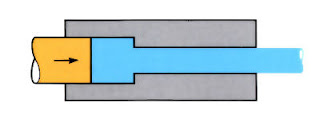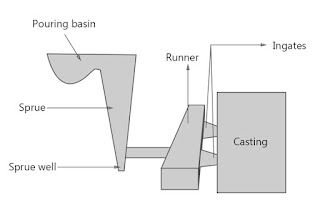## Posts

### Transport Phenomena - short answer questions from AMIE exams (Summer 2019)Answer the following in brief (10 x 2) What are the factors on which viscosity depends? The viscosity of the liquid increases if the density of the liquid increases.  We know that the density of the liquid decreases as the temperature increases and hence viscosity also decreases. So we can say that the viscosity of liquid depends on both density and temperature both.  Differentiate between free and wall turbulence. Turbulence can be generated in two ways: (1) by friction forces at solid walls (or surfaces)  and (2) by the flow of fluid layers with different velocities past the other. These two types  of turbulence are, respectively, known as  wall turbulence and free turbulence. What is ‘The Von Karman analogy? Von Karman extended Prandtl’s analogy by separating the flow field into three distinct layers: a viscous sublayer, a buffer layer, and a turbulent core. In the buffer layer, molecular and eddy diffusivities are assumed to be of the same order of magnitude. The physical significa

### Transport Phenomena - short answer questions from AMIE exams (Winter 2019)Answer the following (20 marks) Explain frictional losses due to sudden expansion and contraction with necessary equations. Head Loss Due to Sudden Contraction in Pipe h c  = 0.5 (V 2 /2g) Head Loss Due to Sudden Expansion in Pipe h e  = (V 1  - V 2 ) 2 /2 Write a short note on Creeping flow and Couette flow. Creeping flow.   Stokes flow also named creeping flow or creeping motion, is a type of fluid flow where advective inertial forces are small compared with viscous forces. The Reynolds number is low, i.e. Re << 1. This is a typical situation in flows where the fluid velocities are very slow, and the viscosities are very large. Couette flow.  In fluid dynamics, Couette flow is the flow of a viscous fluid in the space between two surfaces, one of which is moving tangentially relative to the other. The relative motion of the surfaces imposes shear stress on the fluid and induces flow.  What is Reynolds's analogy? Reynolds's analogy gives the interrelationship between flu

### Transport Phenomena - short answer questions from AMIE exams (Summer 2020)Answer the following in brief (10 x 2) Reynolds analogy Reynolds's analogy gives the interrelationship between fluid friction and newton’s law of viscosity. The Reynolds analogy is given by, N u /R e P r  = S t  = C f /2 where N u  = Local Nusselt number, R e  = Local Reynolds number, S t  = Local Stanton number, P r  = Prandtl number, C f  = Skin friction coefficient Stefan-Boltzmann law Stefan-Boltzmann law states that the total radiant heat power emitted from a surface is proportional to the fourth power of its absolute temperature. where  σ is Stefan’s constant = 5.67 × 10 -8  W/m 2  k 4 Langevin equation Consider a large particle (the Brownian particle) immersed in a fluid of much smaller particles (atoms). Comparison between turbulent thermal conductivity and turbulent viscosity Fourier’s law  states that the negative gradient of temperature and the time rate of heat transfer is proportional to the area at right angles of that gradient through which the heat flows. Fourier’s

### Transport Phenomena - short answer questions from AMIE exams (Winter 2020)Answer the following briefly (20 marks) Dependence of boundary thickness with the distance x from x = 0 for a flat plate. The thickness of the boundary layer increases as the square root of the distance from the leading edge of the plate. Express Nu as the ratio of two temperature gradients. The physical significance of St; Ra. Stanton number indicates the degree of amount of heat delivered by the fluid when there is heat transfer between solid surface and fluid. The greater the Stanton number is, the more effectively heat is transferred.  Rayleigh number is defined as the product of Grashof number and Prandtl number. The rayleigh number is denoted by the symbol Ra. Rayleigh number also to find the type of fluid flow, If Ra < 10⁹, then the flow is laminar and If Ra > 10⁹ then the flow is turbulent. Momentum equation (one-directional) Similarities of kinematic viscosity and mass diffusivity. The kinematic viscosity is also referred to as the momentum diffusivity of the fluid, i.

### Transport Phenomena - short answer question from AMIE exams (Summer 2021)

Answer the following in brief (10 x 2) Define free turbulence and wall turbulence. Turbulence can be generated in two ways: (1) by friction forces at solid walls (or surfaces)  and (2) by the flow of fluid layers with different velocities past the other. These two types  of turbulence are, respectively, known as wall turbulence and free turbulence. Give units of mass diffusivity and kinematic viscosity. The unit of both is m²/s. Differentiate between laminar flow and turbulent flow. Laminar flows are smooth and streamlined, whereas turbulent flows are irregular and chaotic.  A low Reynolds number (< 2000) indicates laminar flow while a high Reynolds number indicates turbulent flow. The flow behaviour drastically changes if it is laminar vs. turbulent. Write expressions for Fourier’s law of heat conduction and the one-dimensional form of Fick’s law of diffusion. Fourier’s law states that the negative gradient of temperature and the time rate of heat transfer is proportional to the ar

### Manufacturing Science - short answer questions from AMIE exams (Winter 2016)Answer the following (10 x 2) Negative rake angles generally are preferred for ceramic, diamond and cubic boron-nitride tools. Why? Although hard and strong in compression, these materials are brittle and relatively weak in tension. Consequently, negative rake angles (which indicate the larger included angle of the tooltip) are preferred mainly because of the lower tendency to cause tensile stresses and chipping of the tools. If a drill bit is intended only for woodworking applications, what material is it most likely to be made from? Because of economic considerations, woodworking tools are typically made of carbon steel, with some degree of hardening by heat treatment. Tool life can be almost infinite at low cutting speeds. Would you then recommend that all machining be done at low speeds? This reason alone would not justify using low cutting speeds. Low cutting speeds remove less material in a given time which, unless otherwise justified, would be economically undesirable. Lower cut

### Manufacturing Science - short answer questions from AMIE exams (Summer 2017)Answer the following (2 x 10) Tool life can be almost infinite at low cutting speeds. Would you then recommend that all matching be done at low speeds? Explain. This reason alone would not justify using low cutting speeds. Low cutting speeds remove less material in a given time which, unless otherwise justified, would be economically undesirable. Lower cutting speeds also often lead to the formation of a built-up edge and discontinuous chips, thus affecting the surface finish. Which tool material properties are suitable for interrupted cutting operations? Why? In interrupted cutting operations, it is desirable to have tools with a high impact strength and toughness. The tool materials which have the best impact strength are high-speed steels, and to a lesser extent, cast alloys and carbides. Therefore, one would prefer to use high-speed steels and carbides in interrupted cutting operations. In addition, in these operations, the tool is constantly heated and reheated. It is therefore de